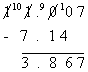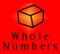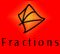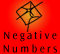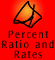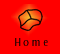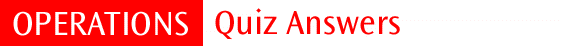| Meaning and models | Key ideas |
| Addition | Subtraction | Multiplication | Division | Mixed operations | Revision |

 1 1.5 2 0.063 3 0.0008 4 6.63 5 1.611

Extra questions:

1.The place values to the right of the decimal point go tenths, hundredths, thousandths (moving from left to right). Hence, the digit in the thousandths column is 2.

2.The 9 is in the second place to the right of the decimal point, so the 9 is in the hundredths place.

3. 0.15 is 15 hundredths so we need to shade 15 rectangles out of the one hundred rectangles.

4. 0.2 is 2 tenths. This means we shade 2 rectangles out of every ten rectangles. There are 100 rectangles here so we shade 20 rectangles

5. One tenth more than four tenths is five tenths. Five tenths is written 0.5

6. Three is the number in the hundredths place in 0.837. Two hundredths less than three hundredths is one hundredth. So, two hundredths less than 0.837 is 0.817

7. The smallest number would have zero tenths and four hundredths, i.e. (.04). Usually this number would be written as 0.04

8. You would have eaten three pieces out of ten, i.e. three tenths. This is written as 0.3

9. Three pieces out of one thousand are lost, i.e. three thousandths. This can be written as 0.003

10. There are 100 hundredths in one and one hundredth in .01. Hence, there are 101 hundredths in 1.01

11. 0.7 is seven tenths. 0.0007 is seven tens of thousandths. Tenths are bigger than tens of thousandths. Hence, 0.7 is bigger than 0.0007

12. 0.9 is nine tenths which equals13. 0.003 is three thousandths which equals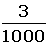14. Here we are counting in tenths. 0.1, 0.2, 0.3, 0.4. The missing number is 0.3

15. Here we are counting in three hundredths. 0.27, 0.30, 0.33, 0.36. The missing numbers are 0.33 and 0.36

16. Caitlin lives four tenths of a kilometre from the school. Jason lives three tenths and seven hundredths of a kilometre from the school. Since four tenths is bigger than three tenths Caitlin lives further from the school.

17. Joan is 1.5 metres and another three hundredths of a metre more so Joan is taller.

18. Three litres and 56 hundredths of a litre is 3.56 litres. Three litres and four tenths of a litre is 3.4 litres. Since 4 tenths is less than 5 tenths 3.4 litres is smaller.

19. Five ten thousandths of the people are over seventy. This is 0.0005

20. (a) Twelve out of 100 is twelve hundredths. This is one tenth and two hundredths.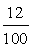(b) Twelve out of 100 is twelve hundredths. This is one tenth and two hundredths.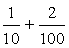(c) Twelve hundredths equals 0.12

 1. (a) 297.5 297.518 is between 297.5 and 297.6, but nearer to 297.5 (b) 300 297.518 is between 200 and 300, but nearer to 300 than 200 (c) 297.52 297.518 is between 297.51 and 297.52, but nearer to 297.52 2. (a) 0.867 0.86725 is nearer to 0.867 than to 0.868 (b) 1 0.8675 is nearer to 1 than to 0 (c) 0.87 0.86725 is nearer to 0.87 than to 0.86 (d) 0.8673 0.86725 is exactly half way between 0.8672 and 0.8673, it is usual to move it up to the larger number

 1.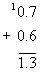2.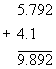3.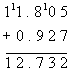4.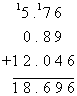Extra questions:

 1.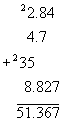2. More means add.3. Distance traveled altogether equals the sum of the three distances traveled.4. Milk drunk Monday = 0.875 L Milk drunk Tuesday = 0.875 + 0.26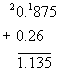Total milk drunk in 2 days = 0.875 + 1.135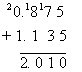Rose drank 2.01L of milk in the two days. 5. Total length of string = 1.987 + 2.03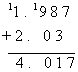The total length of the string is 4.017 m 6. The certain number must be 12.9024 more than 1.839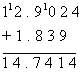The certain number is 14.7414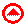1.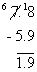2.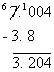3.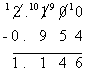4.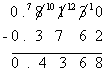Extra questions:

 1.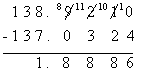2.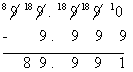3. The certain number is 19.723 less than 271.65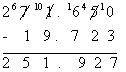4.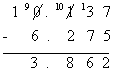5.Meg is 10.4 cm taller than Abdul 6.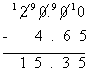I will get \$15.35 change1. (a) 2914.2 4857 x 0.6 is one tenth of 4857 x 6 (b) 29.142 48.57 is one hundredth of one tenth of 4857 x 6 (c) 0.29142 485.7 x 0.6 is one tenth of one thousandth of 4857 x 6 2. 11.43 15 x 762 = 11430, so 1.5 x 7.62 is one tenth of one hundredth of 11430 3. 0.024 4 x 6 = 24, so 0.4 x 6 is one tenth of 24, and 0.4 x 0.06 is one hundredth of one tenth of 24 4. 0.00010 2 x 5 = 10. Do not lose the final zero if you are counting the decimal places

Extra questions:

 1.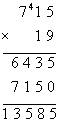7.15 has 2 digits after the decimal and 1.9 has 1 digit after the decimal. This is a total of 3 digits after the decimals, so there must be 3 digits after the decimal in the answer Therefore 7.15 x 1.9 = 13.585 2.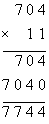7.04 has 2 digits after the decimal and 1.1 has 1 digit after the decimal. This is a total of 3 digits after the decimals, so there must be 3 digits after the decimal in the answer Therefore 7.04 x 1.1 = 7.744 3. Steve strides 84.6 cm 200 times Distance traveled = 84.6 x 200 = 84.6 x 100 x 2 = 8460 x 2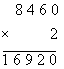Distance traveled by Steve = 16 920 cm. 4. Length of front garden bed = 156 x 4.3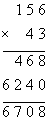156 has no digits after the decimal and 4.3 has 1 digit after the decimal. This is a total of 1 digit after the decimals, so there must be 1 digit after the decimal in the answer Therefore 156 x4.3 = 670.8 Length of front garden bed = 670.8 cm 5. The statement is false. Multiplying 9.7634 by a decimal less than 1 will give a smaller answer than 9.7634.1. (a) 158.95 794.75 ÷ 5 is one hundredth of 79475 ÷ 5 (b) 158.95 79.475 ÷ 0.5 is ten times one thousandth of 79475 ÷ 5 (c) 158.95 7.9475 ÷ 0.05 is one hundred times a ten thousandth of 79475 ÷ 5 (d) 1589.5 7.9475 ÷ 0.005 is one thousand times a ten thousandth of 79475 ÷ 5 2. 90 45 ÷ 0.5 is ten times 45 ÷ 5 3. 3.12 6.864 ÷ 2.2 is ten times a thousandth of

Extra questions:

 1. 14.606 ÷ 0.4 = 146.06 ÷ 4 (make the divisor a whole number)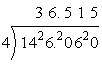2. 28.941 ÷ 0.03 = 2894.1 ÷ 33. 35.4 ÷ 60 = 35.4 ÷ 10 ÷ 6 = 3.54 ÷ 6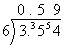4. 57.6 divided into 12 equal pieces = 57.6 ÷ 12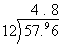Each of Sean’s pieces of ribbon will be 4.8 cm long 5. 18.4 mls how many 0.8’s? This is 18.4 ÷ 0.8 18.4 ÷ 0.8 = 184 ÷ 8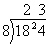There are 23 doses. 6. \$328.86 shared between 9 people. This is 328.86 ÷ 9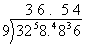Each person will recieve \$36.541. 0.707, 0.72, 0.07, 0.207

The numbers have 7 tenths, 7 tenths, zero tenths and 2 tenths respectively. Hence, 0.07 is the smallest of the numbers. Out of 0.707 and 0.72, they have zero hundredths and 2 hundredths respectively. Hence, 0.72 is the largest of all the numbers given.

The sum of the biggest and least = 0.72 + 0.07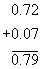2. Each of the given decimals has 7 tenths. Now look at the hundredths column. 0.7031, 0.7003 and 0.701 have zero hundredths, whereas 0.731 has 3 hundredths. Hence, 0.731 is not the smallest. Now look at the thousandths column.0.7031 has 3 thousandths, 0.7003 has zero thousandths and 0.701 has 1 thousandth. Hence, 0.7003 is the smallest of the given decimals.

3. The 7 is in the tenths place in 89.765

The digit after this is greater than 5 so round up. 89.8

4. Combined distance = 9 lots of 24.3 km = 9 x 24.3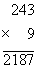24.3 has 1 digit after the decimal point and 9 has no digits after the decimal point. This is a total of 1 digit after the decimals, so there must be 1 digit after the decimal in the answer

Therefore 24.3 x 9 = 218.7

The combined distance traveled is 218.7 km

5. Start with the largest of the given numbers which is 4.8

The smaller the number you divide by the larger the resulting number, so divide by the smallest number which is 0.8. The smaller the number you subtract from any number, the larger the answer, so subtract the next smallest number which is 1.2

The largest number is 4.8 ÷ 0.8 - 1.2 = 4.8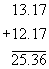Difference means take the smaller from the larger number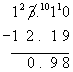Product means multiply.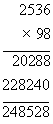25.36 has 2 digits after the decimal point and 0.98 has 2 digits after the decimal point. This is a total of 4 digits after the decimals, so there must be 4 digits after the decimal in the answer

Therefore 25.36 x 0.98 = 24.8528

7.Cost of bread = 2.33 x 4

Cost of milk = 1.85 x 2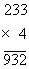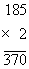2.33 has 2 digits after the decimal point and 4 has no digits after the decimal point. This is a total of 2 digits after the decimals, so there must be 2 digits after the decimal in the answer

Therefore 2.33 x 4 = 9.32

1.85 has 2 digits after the decimal point and 2 has no digits after the decimal point. This is a total of 2 digits after the decimals, so there must be 2 digits after the decimal in the answer

Therefore 1.85 x 2 = 3.70

The total cost of bread and milk = 9.32 + 3.70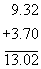The total cost of bread and milk = \$13.021.2. In 0.6751 the 7 is in the hundredths column. The next digit is 5, hence we round the 7 up to 8, 0.6751 to the nearest hundredth is 0.68 3. 8 x 5 = 40 In 0.8 x 5 there is a total of one digit after the decimal point, so there must be one digit after the decimal point in the answer So 0.8 x 5 = 4.0 = 4 4.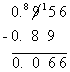5. 15.35 ÷ 0.5 Make the divisor a whole number: 0.5 x 10 = 5 So 15.35 ÷ 0.5 = 153.5 ÷ 5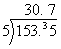6. 0.81 ÷ 90 = 0.81 ÷ 10 ÷ 9 = 0.081 ÷ 9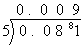7. 70 x 5 = 350 In 70 x 0.05 there are a total of two digits after the decimal point, so there must e teo digits after the decimal point in the answer. So 70 x 0.05 = 3.50 = 3.5 8.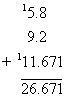9. In 0.9172, the 0 is in the ones position. The next digit, 9 is greater than 5 so round the 0 up. Hence, 0.9172 to the nearest one is 1. 10.